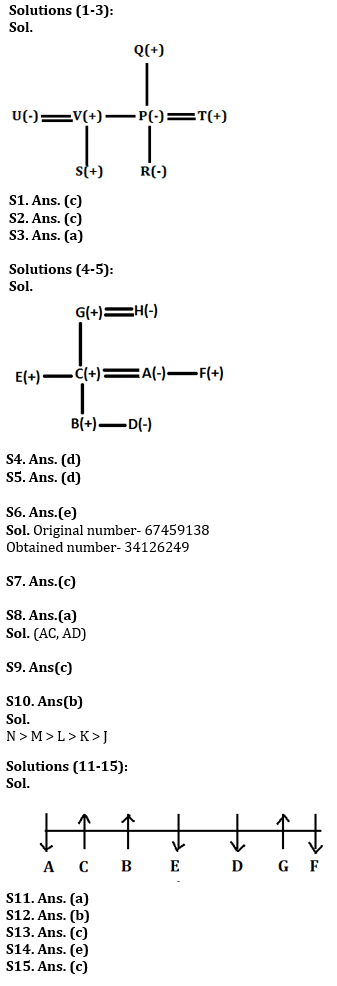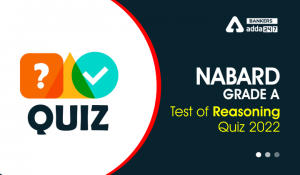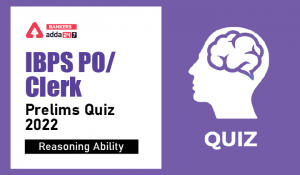Latest Banking jobs   »

# Reasoning Ability Quiz For IBPS Clerk Prelims 2022- 17th July

Directions (1-3): Study the information carefully and answer the questions given below.
There are seven persons- P, Q, R, S, T, U and V in a family. There are two married couples and three generations in this family. Q is father-in-law of T. P is mother of R. S is nephew of T. R is niece of U. V is brother-in-law of T. U is mother of S.

Q1. Who among the following is father of R?
(a) P
(b) V
(c) T
(d) Q
(e) None of these

Q2. Who among the following are children of Q?
(a) T, U
(b) S, R
(c) V, P
(d) T, S
(e) None of these

Q3. How is U related to Q?
(a) Daughter-in-law
(b) Father-in-law
(c) Daughter
(d) Mother-in-law
(e) None of these

Directions (4-5): Study the following information and answer the questions given below.
A family consists of eight members A, B, C, D, E, F, G and H. B is the son of C but C is not the mother of B. A and C are married couple. E is the brother of C. D is the daughter of A and F is the brother of A. G is the father-in-law of A. B is grandson of H. G is a married man.

Q4. How is H related to A?
(a) father
(b) father-in-law
(c) Sister
(d) mother-in-law
(e) mother

Q5. How is D related to F?
(a) Son
(b)Uncle
(c) Daughter
(d) Niece
(e) Nephew

Q6. If in the number 67459138, 3 is subtracted to first five digit of the number and 1 is added to rest of the digits then how many digits are repeating in the number thus formed?
(a) Four
(b) One
(c) None
(d) three
(e) Two

Q7. Which of the following elements should come in a place of ‘?’?
GJ11 MO16 RT21 ?
(a) WX26
(b) VX25
(c) WY26
(d) VY26
(e) None of these

Q8. How many pairs of letters are there in the word “ENDURANCE” each of which have as many letters between them in the word as they have between them in the English alphabetical series?
(a) Two
(b) One
(c) Three
(d) More than three
(e) None

Q9. Ravi is 22nd from the left end of a row and Hunny is 32th from the right end of row. If they interchanged their positions then Ravi ranks become 21 from left end. How many persons sit between them?
(a) One
(b) Two
(c) None
(d) Three
(e) None of these

Q10. Among J, K, L, M and N, each of them is different weight. L’s weight is more than K. M’s weight is more than J and less than N. K is not the lightest person. M is not lighter than L. Who among them is the third heaviest?
(a) J
(b) L
(c) K
(d) M
(e) N

Directions (11-15): Study the following information carefully and answer the questions given below:
Seven persons A, B, C, D, E, F, and G sit in a row some are facing north and some are facing south direction but not necessarily in the same order. The number of persons facing south is more than the number of persons facing north direction. A sits immediate left of C and A sits at one of the extreme ends of the row. Number of persons sitting to the left of E is same as the number of persons sitting to the right of E. A sits to the right of E. One person sits between D and F and F is not immediate neighbour of E. G sits to the right of C but not immediate right. D is facing the same direction as E. A and C are facing opposite direction to each other. The persons sitting at the end are facing the same direction. The person who sits at the immediate right of E is facing north direction. B and G is facing the same direction.

Q11.Who among the following person sits exactly between C and G?
(a) E
(b) A
(c) D
(d) F
(e) None of these

Q12.What is the position of D with respect to B?
(a) Immediate left
(b) Second to the right
(c) Second to the left
(d) Immediate right
(e) None of these

Q13. How many persons sit between G and F?
(a) One
(b) Two
(c) None
(d) Three
(e) None of these

Q14.Four of the following five are alike in a certain way and hence form a group find the one which does not belong to that group?
(a) A
(b) E
(c) D
(d) F
(e) G

Q15. If B and F interchange their position then who sits immediate right of B?
(a) C
(b) D
(c) G
(d) A
(e) None of these

Solutions#### Congratulations!Incorrect details? Fill the form again here

•Reasoning Ability Quiz For IBPS RRB PO C...
•Reasoning Ability Quiz For NABARD Grade ...
•Reasoning Ability Quiz For IBPS Clerk/PO...
•Reasoning Ability Quiz For IBPS RRB PO C...
•Reasoning Ability Quiz For IBPS Clerk Pr...
•Reasoning Ability Quiz For IBPS RRB PO C...